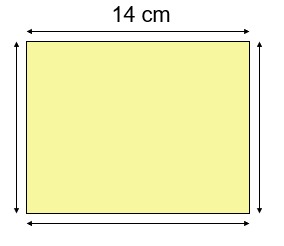# Perimeter of Square

Perimeter of Square is equal to four times of its side.

Perimeter of Square = 4 x Length of each side

Question 1

Find the perimeter of a square, each of whose side is 14 cm ?

Explanation:Square is a four sided polygon having all four sides equal in length.

Given:
Side = 14 cm
Perimeter of a square = ( 4 x Length of each side ) units
= ( 4 x 14 ) cm
= 56 cm

Question 2

Find the perimeter of a square, each of whose side is 18 cm?

Explanation:

Square is a four-sided polygon having all four sides equal in length.
Given:
Side = 18 cm
Perimeter of square = ( 4 x Length of each side ) units
= ( 4 x 18 ) cm
= 72 cm

Question 3

Find the perimeter of a square, each of whose side is 20 cm?

Explanation:

Square is a four-sided polygon having all four sides equal in length.
Given:
Side = 20 cm
Perimeter of square = ( 4 x Length of each side ) units
= ( 4 x 20 ) cm
= 80 cm

Question 4

Find the perimeter of a square, each of whose side is 25 cm?

Explanation:

Square is a four-sided polygon having all four sides equal in length.
Given:
Side = 25 cm
Perimeter of square = ( 4 x Length of each side ) units
= ( 4 x 25 ) cm
= 100 cm

Question 5

Find the perimeter of a square, each of whose side is 5.6 cm?

Explanation:

Square is a four-sided polygon having all four sides equal in length.
Given:
Side = 5.6 cm
Perimeter of square = ( 4 x Length of each side ) units
= ( 4 x 5.6 ) cm
= 22.4 cm

Question 6

Find the perimeter of a square, each of whose side is 7.6 cm?

Explanation:

Square is a four-sided polygon having all four sides equal in length.
Given:
Side = 7.6 cm
Perimeter of square = ( 4 x Length of each side ) units
= ( 4 x 7.6 ) cm
= 30.4 cm